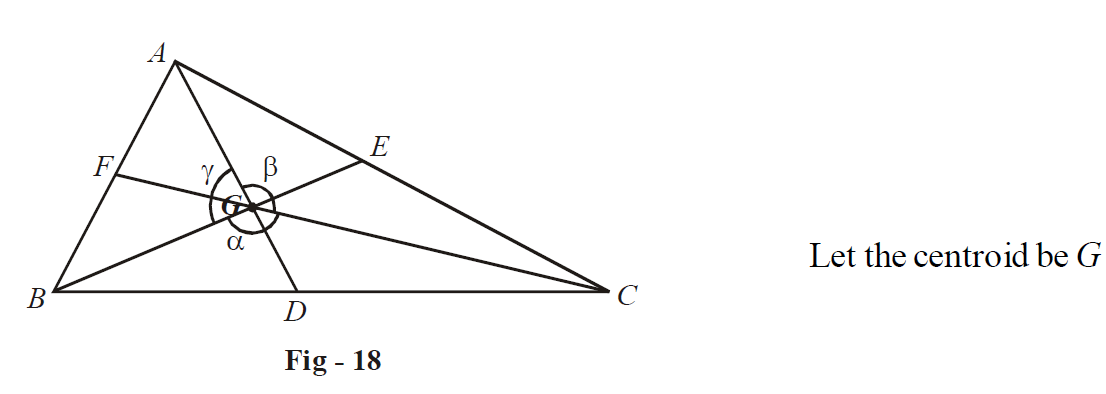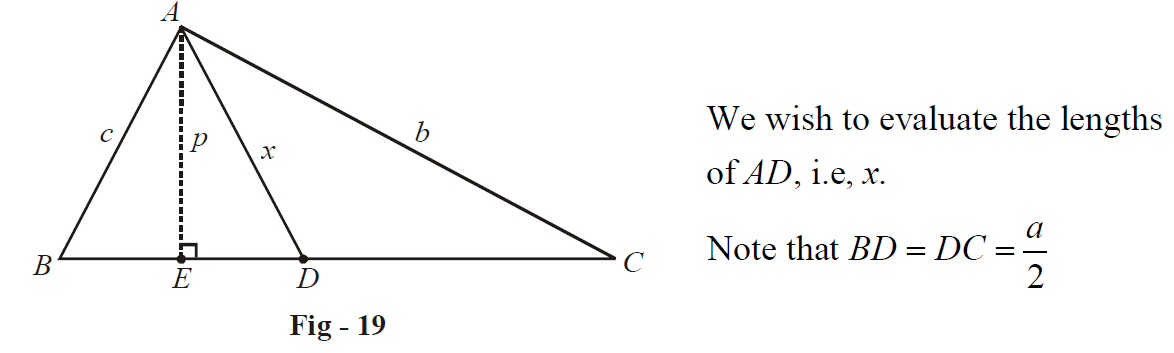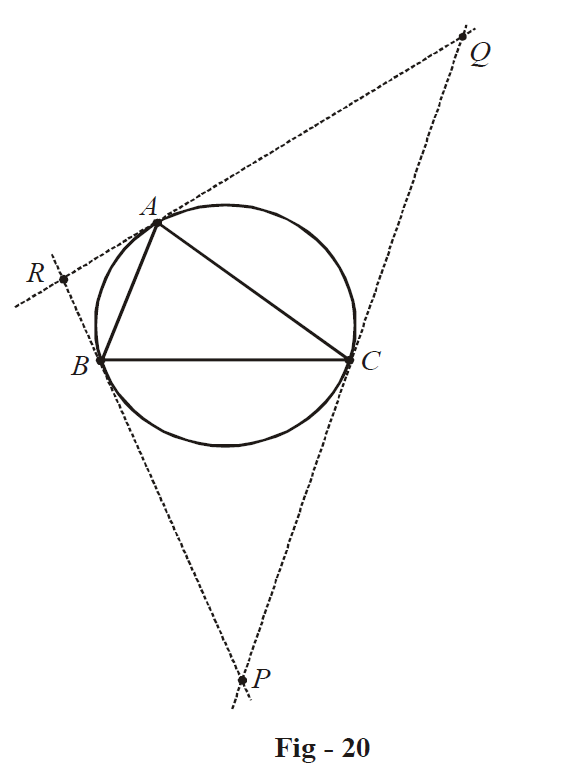# Examples on Cosine Rule Set 3

Go back to  'Trigonometry'

Example - 48

Suppose that the medians of a  $$\Delta ABC$$ make angles  $$\alpha ,\;\beta ,\;\gamma$$  with each other. Find the value of  $$S = \cot \alpha + \cot \beta + \cot \gamma + \cot A + \cot B + \cot C$$

Solution:To proceed, we first evaluate the lengths of the medians of the triangle in terms of the sides. Consider the following diagram:By the pythagoras theorem,

${x^2} = {p^2} + D{E^2} = \frac{1}{2}\left\{ {{p^2} + {p^2} + 2D{E^2}} \right\}$

Note that  \begin{align}{p^2} = {c^2} - B{E^2} = {b^2} - C{E^2},\;{\text{and}}\;DE = \frac{a}{2} - BE = CE - \frac{a}{2}\end{align}  .

Thus,

\begin{align}&{x^2} = \frac{1}{2}\left\{ {({c^2} - B{E^2}) + ({b^2} - C{E^2}) + 2\left( {\frac{a}{2} - BE} \right)\left( {CE - \frac{a}{2}} \right)} \right\}\\ \,\,\,\,\,\,\, &\quad= \frac{1}{2}\left\{ {{b^2} + {c^2} - (B{E^2} + C{E^2}) - \frac{{{a^2}}}{2} + a(CE + BE) - 2BE \cdot CE} \right\} \\ \,\,\,\,\,\,\, &\quad= \frac{1}{2}\left\{ {{b^2} + {c^2} - \frac{{{a^2}}}{2} + {a^2} - {{(BE + CE)}^2}} \right\} \\ \,\,\,\,\,\,\, &\quad= \frac{1}{2}\left\{ {{b^2} + {c^2} - \frac{{{a^2}}}{2}} \right\} = \frac{{2{b^2} + 2{c^2} - {a^2}}}{4} \qquad\qquad...(1) \end{align}

Similar expressions will hold for the other medians. Now, we return to the original problem.

Applying the cosine rule in $$\Delta BGC$$ , we have

$B{C^2} = {a^2} = B{G^2} + C{G^2} - 2BG \cdot CG \cdot \cos \alpha$

Now, since G is the centroid, we have  \begin{align}BG = \frac{2}{3}BE,\;CG = \frac{2}{3}CF,\end{align}  so that

${a^2} = \frac{4}{9}B{E^2} + \frac{4}{9}C{F^2} - \frac{8}{9}BE \cdot CF \cdot \cos \alpha \,\,\,\,\,\,\,\,\,\,\,\,\,\,\,\,\,\,\,\,\, \ldots \left( 2 \right)$

Also, if the area of  $$\Delta BGC$$  is   $${\Delta _1}$$ , then

${\Delta _1} = \frac{1}{2} \cdot BG \cdot CG \cdot \sin \alpha = \frac{2}{9}BE \cdot CF \cdot \sin \alpha$

But  $${\Delta _1}$$  is one thirds of the total area  $$\Delta$$  of  $$\Delta ABC$$ (why?), so that

$BE \cdot CF = \frac{{3\Delta }}{{2\sin \alpha }}\,\,\,\,\,\,\,\,\,\,\,\,\,\,\,\,\,\,\,\,\, \ldots \left( 3 \right)$

Using (3) in (2), we have

\begin{align} &\qquad\quad \;\;\,{a^2} = \frac{4}{9}\left( {B{E^2} + C{F^2}} \right) - \frac{{4\Delta }}{3}\cot \alpha \\ & \Rightarrow \quad \cot \alpha = \frac{{4(B{E^2} + C{F^2}) - 9{a^2}}}{{12\Delta }} \\ \end{align}

Similar expressions hold for  $$\cot \beta \;{\text{and}}\;\cot \gamma$$  . Summing the expressions for the three ‘cot’s, and using the result of (1) and simiplfying, we have

\begin{align} \cot \alpha + \cot \beta + \cot \gamma = \frac{{8(A{D^2} + B{E^2} + C{F^2}) - 9({a^2} + {b^2} + {c^2})}}{{12\Delta }} \\ \,\,\,\,\,\,\,\,\,\,\, = \frac{{ - ({a^2} + {b^2} + {c^2})}}{{4\Delta }}\,\,\,\,\,\,\,\,\,\,\,\,\,\,\,\,\,\,\,\,\,\,\,\,\,\,\,\,\,\,\,\,\,\,\,\,\,\,\,\,\,\,\,\, \ldots \left( 4 \right) \\ \end{align}

Also, if you refer to Example – 46, you’ll note that we’ve already calculated the sum  $$\cot A + \cot B + \cot C$$  :

$\cot A + \cot B + \cot C = \frac{{({a^2} + {b^2} + {c^2})}}{{4\Delta }}\,\,\,\,\,\,\,\,\,\,\,\,\,\,\,\,\,\,\,\,\,\,\,\,\,\,\,\,\,\,\,\,\,\,\,\,\, \ldots \left( 5 \right)$

From (4) and (5), the required sum is zero.

Example - 49

Consider a triangle $$\Delta ABC$$ . Let  $$\Psi$$ be the circumcircle of  $$\Delta ABC$$ . At each of the three points A, B, C, tangents are drawn to $$\Psi$$ to form another triangle, $$\Delta PQR$$  . Find the sides of  $$\Delta PQR$$ .

Solution:Let us evaluate the side PQ.

Note that

$\angle PCB = \angle PBC = \angle A\qquad\qquad(why?)$

so that

$\angle P = \pi - 2\angle A$

Applying the sine rule in  $$\Delta PBC$$ , we have

\begin{align} & \qquad \frac{{PB}}{{\sin A}} = \frac{{PC}}{{\sin A}} = \frac{{BC}}{{\sin 2A}} \\ &\Rightarrow \quad PC = \frac{{BC\sin A}}{{\sin 2A}} = \frac{a}{{2\cos A}} \\ \end{align}

Similarly, \begin{align}QC = \frac{b}{{2\cos B}}\end{align}  . Thus,

\begin{align}&PQ = PC + CQ = \frac{a}{{2\cos A}} + \frac{b}{{2\cos B}} = \frac{1}{2}\frac{{(a\cos B + b\cos A)}}{{\cos A\cos B}} \\ &\qquad\qquad\qquad\qquad\qquad\qquad\qquad\qquad\;= \frac{{\cos C}}{{2\cos A\cos B}} \\ \end{align}

Similar expressions will hold for QR and RP.